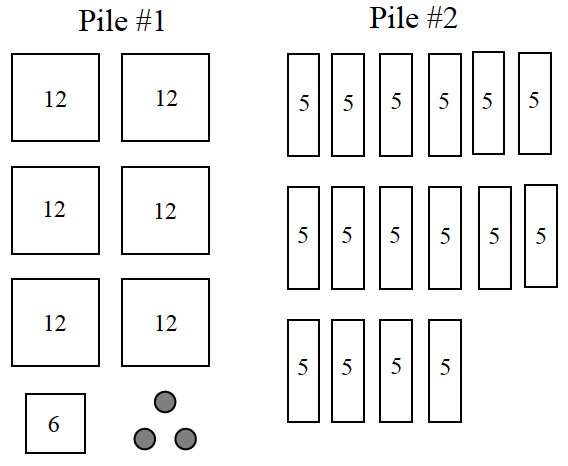### Home > CC1 > Chapter Ch1 > Lesson 1.2.2 > Problem1-58

1-58.

The diagrams at right represent piles of pennies. Homework Help ✎

1. Which pile has more pennies? Explain your reasoning.

Try calculating the sum of each pile.

Don't forget to state which pile has more!

Pile #1 has $81$ pennies and Pile #2 has $80$ pennies.

2. Write two different numerical expressions to represent the number of pennies in each pile.

What different ways can you calculate the sums of the piles?

For instance: $\text{Pile #1} = (6)(12) + 6 + 3$

3. Write a number comparison statement (using $>$ , $<$ , or $=$ ) to show if the number of pennies in one pile is greater than the other or if they are the same.

Remember:
$>$ means that the number on the left is greater than the number on the right and $<$ means that the number on the left is less than the number on the right.